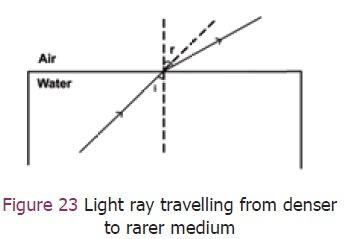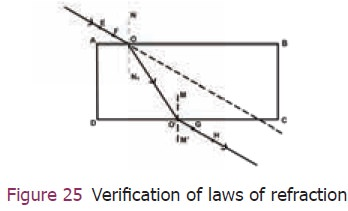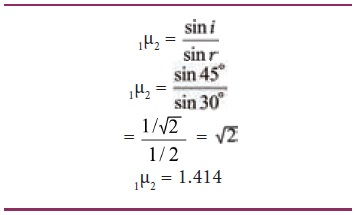Home | | Science 9th std | Speed of light

# Speed of light

In early seventeenth century, the Italian scientist Galileo Galilee (1564‒1642) tried to measure the speed of light as it travelled from a lantern on a hill top about a mile (1.6 km) away from where he stood.

Speed of light

In early seventeenth century, the Italian scientist Galileo Galilee (1564‒1642) tried to measure the speed of light as it travelled from a lantern on a hill top about a mile (1.6 km) away from where he stood. His attempt was bound to fail, because he had no accurate clocks or timing instruments.

In 1665 the Danish astronomer Ole Roemer first estimated the speed of light by observing one of the twelve moons of the planet Jupiter. As these moons travel around the planet, at a set speed, it would take 42 hours to revolve around Jupiter. Roemer made a time schedule of the eclipses for the whole year. He made first observation in June and second observation in December. Roemer estimated the speed of light to be about 220,000 km per second.

In 1849 the first land based estimate was made by Armand Fizeau. Today the speed of light in vacuum is known to be almost exactly 300,000 km per second.

## 1. Refraction of light## 2. Cause of refraction

Light rays get deviated from their original path while entering from one transparent medium to another medium of different optical density. This deviation (change in direction) in the path of light is due to the change in velocity of light in the different medium. The velocity of light depends on the nature of the medium in which it travels. Velocity of light in a rarer medium (low optical density) is more than in a denser medium (high optical density).

## 3. Refraction of light from a plane transparent surface

When a ray of light travels from optically rarer medium to optically denser medium, it bends towards the normal. (Figure 22)When a ray of light travels from an optically denser medium to an optically rarer medium it bends away from the normal. (Figure 23)A ray of light incident normally on a denser medium, goes without any deviation. (Figure 24).## 4. The laws of refraction of light

The incident ray, the refracted ray and the normal to the interface of two transparent media at the point of incidence, all lie in the same plane.

The ratio of the sine of the angle of incidence to the sine of the angle of refraction is a constant for a light of a given colour and for the given pair of media. is law is also known as Snell’s law of refraction.

If i is the angle of incidence and r is the angle of refraction, then

Sin i / sin r = constant

This constant is called the refractive index of the second medium with respect to the first medium. It is generally represented by the Greek letter, 1µ2 (mew).

Note: The refractive index has no unit as it is the ratio of two similar quantities.

## 5. Verification of la  s of refraction## 6. Speed of light in different media

Light has the maximum speed in vacuum and it travels with different speeds in different media. The speed of light in some media is given below.Note: The refractive index of a medium is also defined in terms of speed of light in different media### Sample problem 4

The speed of light in air is 3 × 108 ms-1 and in glass it is 2 × 108 ms-1 what is the refractive index of glass.### Sample problem 5

Light travels from a rarer medium to a denser medium. The angles of incidence and refraction are respectively 45° and 30°. Calculate the refractive index of the second medium with respect to the rst medium.

Angle of incidence i = 45°

Angle of refraction r = 30°Study Material, Lecturing Notes, Assignment, Reference, Wiki description explanation, brief detail
9th Science : Light : Speed of light |